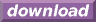#Calcute: freeware calculator

## Hyperbolic Arctangent (hyperbolic arc-tangent, hyperbolic inverse tangent, atanh)

The hyperbolic arc-tangent is the inverse of the hyperbolic tangent function. Calcute implements it with the atanh function. The inverse hyperbolic tangent of a value x is the value y for which the hyperbolic tangent of y is x. In other words if y = atanh(x) then x = tanh(y).

```atanh(0.5)
0.549306144334
```

The hyperbolic arc-tangent function is only defined in the open range (-1, +1). This corresponds to the output range of the hyperbolic tangent function.

In some mathematical texts and hand-held calculators, the notation tanh-1 is sometimes used to represent the hyperbolic arc-tangent function. This is an unfortunate notational choice since it could also stand for the multiplicative inverse 1/tanh which is a different function.

Yet another term also used to represent the inverse hyperbolic tangent function is "arctanh".